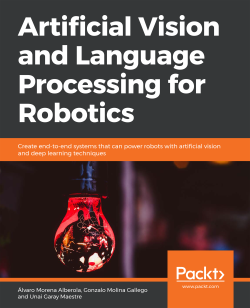### Artificial Vision and Language Processing for Robotics

By Álvaro Morena Alberola , Gonzalo Molina Gallego , Unai Garay Maestre
• Constantly updated with 100+ new titles each month
• Breadth and depth in over 1,000+ technologies

Artificial Vision and Language Processing for Robotics begins by discussing the theory behind robots. You'll compare different methods used to work with robots and explore computer vision, its algorithms, and limits. You'll then learn how to control the robot with natural language processing commands. You'll study Word2Vec and GloVe embedding techniques, non-numeric data, recurrent neural network (RNNs), and their advanced models. You'll create a simple Word2Vec model with Keras, as well as build a convolutional neural network (CNN) and improve it with data augmentation and transfer learning. You'll study the ROS and build a conversational agent to manage your robot. You'll also integrate your agent with the ROS and convert an image to text and text to speech. You'll learn to build an object recognition system using a video.

By the end of this book, you'll have the skills you need to build a functional application that can integrate with a ROS to extract useful information about your environment.

Publication date:
April 2019
Publisher
Packt
Pages
356
ISBN
9781838552268

## ChapterÂ 1.Â Fundamentals of Robotics

### Note

Learning Objectives

By the end of this chapter, you will be able to:

• Describe important events in the history of robotics

• Explain the importance of using artificial intelligence, artificial vision and natural language processing

• Classify a robot depending on its goal or function

• Identify the parts of a robot

• Estimate a robot's position using odometry

### Note

This chapter covers the brief history of robotics, classifies different types of robots and its hardware, and explains a way to find a robot's position using odometry.

## Introduction

The robotics sector represents the present and the future of humanity. Currently, there are robots in the industrial sector, in research laboratories, in universities, and even in our homes. The discipline of robotics is continually evolving, which is one of the reasons it is worth studying. Every robot needs someone to program it. Even those based on AI and self-learning need to be given initial goals. Malfunctioning robots need technicians and constant maintenance, and AI-based systems need constant data inputs and monitoring to be effective.

In this book, you will learn and practice lots of interesting techniques, focusing on artificial computer vision, natural language processing, and working with robots and simulators. This will give you a solid basis in some cutting-edge areas of robotics.

## History of Robotics

Robotics stemmed from the need to create intelligent machines to perform tasks that were difficult for humans. But it wasn't called â€œroboticsâ€ at first. The term â€œrobotâ€ was coined by a Czech writer, Karel ÄŒapek, in his work R.U.R. (Rossum's Universal Robots). It is derived from the Czech word robota, which means servitude and is related to forced labor.

ÄŒapek's work became known worldwide, and the term â€œrobotâ€ did too, so much so that this term was later used by the famous teacher and writer Isaac Asimov in his work; he termed robotics as the science that studies robots and their features.

Here you can see a timeline of the important events that have shaped the history of robotics: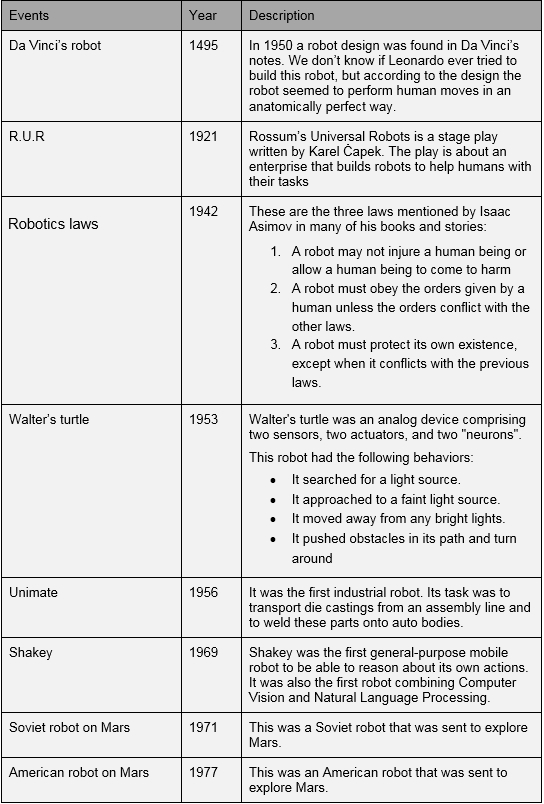Figure 1.1: History of robotics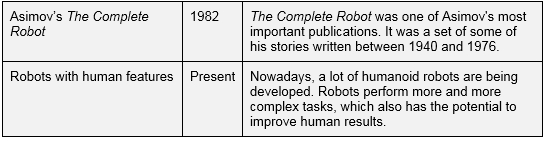Figure 1.2: History of robotics continued

Figures 1.1 and 1.2 give a useful timeline of the beginnings and evolution of robotics.

## Artificial Intelligence

AI refers to a set of algorithms developed with the objective of giving a machine the same capabilities as that of a human. It allows a robot to take its own decisions, interact with people, and recognize objects. This kind of intelligence is present not just in robots, but also in plenty of other applications and systems (even though people may be unaware of it).

There are many real-world products already using this kind of technology. Here's a list of some of them to show you the kind of interesting applications you can build:

• Siri: This is a voice assistant created by Apple, and is included in their phones and tablets. Siri is very useful as it is connected to the internet, allowing it to look up data instantly, send messages, check the weather, and do much more.

• Netflix: Netflix is an online film and TV service. It runs on a very accurate recommendation system that is developed using AI that recommends films to users based on their viewing history. For example, if a user usually watches romantic movies, the system will recommend romantic series and movies.

• Spotify: Spotify is an online music service similar to Netflix. It uses a recommendation system to make accurate song suggestions to users. To do so, it considers songs that the user has previously heard and the kind of music added to the user's library.

• Tesla's self-driving cars: These cars are built using AI that can detect obstacles, people, and even traffic signals to ensure the passengers have a secure ride.

• Pacman: Like almost any other video game, Pacman's enemies are programmed using AI. They use a specific technique that constantly computes the collision distance, taking into account wall boundaries, and they try to trap Pacman. As it is a very simple game, the algorithm is not very complex, but it is a good example that highlights the importance of AI in entertainment.

### Natural Language Processing

Natural Language Processing (NLP) is a specialized field in AI that involves studying the different ways of enabling communication between humans and machines. It is the only technique that can make robots understand and reproduce human language.

If a user uses an application that is supposed to be capable of communicating, the user then expects the application to have a human-like conversation. If the humanoid robot uses badly formed phrases or does not give answers related to the questions, the user's experience wouldn't be good and the robot wouldn't be an attractive buy. This is why it is very important to understand and make good use of NLP in robotics.

Let's have a look at some real-world applications that use NLP:

• Siri: Apple's voice assistant, Siri, uses NLP to understand what the user says and gives back a meaningful response.

• Cortana: This is another voice assistant that was created by Microsoft and is included in the Windows 10 operating system. It works in a similar way to Siri.

• Bixby: Bixby is a part of Samsung that is integrated in the newest Samsung phones, and its user experience is similar to using Siri or Cortana.

### Note

You may be asking which one of these three is the best; however, it depends on each user's likes and dislikes.

• Phone operators: Nowadays, calls to customer services are commonly answered by answering machines. Most of these machines are phone operators that work by receiving a keyword input. Most modern operators are developed using NLP in order to have more realistic conversations with clients over the phone.

• Google Home: Google's virtual home assistant uses NLP to respond to users' questions and to perform given tasks.

### Computer Vision

Computer vision is a commonly used technique in robotics that can use different cameras to simulate the biomechanical three-dimensional movement of the human eye. It can be defined as a set of methods used to acquire, analyze, and process images and transform them into information that can be valuable for a computer. This means that the information gathered is transformed into numerical data, so that the computer can work with it. This will be covered in the chapters ahead.

Here's a list of some real-world examples that use computer vision:

• Autonomous cars: Autonomous cars use computer vision to obtain traffic and environment information and to decide what to do on the basis of this information. For example, the car would stop if it captures a crossing pedestrian in its camera.

• Phone camera applications: Many phone-based camera applications include effects that modify a picture taken using the camera. For example, Instagram allows the user to use filters in real time that modify the image by mapping the user's face to the filter.

• Tennis Hawk-Eye: This is a computer-based vision system used in tennis to track the trajectory of the ball and display its most likely path on the court. It is used to check whether the ball has bounced within the court's boundaries.

### Types of Robots

When talking about AI and NLP, it is important to take a look at real-world robots, because these robots can give you a fair idea of the development and improvement of existing models. But first, let's talk about the different kinds of robots that we can find. Generally, they can be classified as industrial-based robots and service-based robots, which we will discuss in the following sections.

### Industrial Robots

Industrial robots are used in manufacturing processes and don't usually have a human form. In general, they pretty much look like other machines. This is because they are built with the aim of executing a specific industrial task.

### Service Robots

Service robots work, either partially or entirely, in an autonomous manner, and perform useful tasks for humans. These robots can also be further divided into two groups:

• Personal robots: These are commonly used in menial house-cleaning tasks, or in the entertainment industry. This is the kind of machine that people always imagine when discussing robots, and they are often imagined to have human-like features.

• Field robots: These are robots in charge of military and exploratory tasks. They are built with resistant materials because they must withstand harsh sunlight and other external weather agents.

Here you can see some examples of real-world personal robots:

• Sophia: This is a humanoid robot created by Hanson Robotics. It was designed to live with humans and to learn from them.

• Roomba: This is a cleaning robot made by iRobot. It consists of a wheelie circular base that moves around the house while computing the most efficient way to cover the entire area.

• Pepper: Pepper is a social robot designed by SoftBank Robotics. Although it has human form, it doesn't move in a bipedal way. It also has a wheelie base that provides good mobility.

### Hardware and Software of Robots

Just like any other computer system, a robot is composed of hardware and software. The kind of software and hardware the robot has will depend on its purpose and the developers designing it. However, there are a few types of hardware components that are more commonly used in several robots. We will be covering these in this chapter.

First of all, let's look at the three kinds of components that every robot has:

• Control system: The control system is the central component of the robot, which is connected to all other components that are to be controlled. It is usually a microcontroller or a microprocessor, the power of which depends on the robot.

• Actuators: Actuators are a part of the robot that allows it to make changes in the external environment, such as a motor for moving the whole robot or a part of the robot, or a speaker that allows the robot to emit sounds.

• Sensors: These components are in charge of obtaining information so that the robot can use it to have the desired output. This information can be related to the robot's internal status or to its external circumstances. Based on this, the sensors are divided into the following types:

• Internal sensors: Most of these are used for the measuring position of the robot, so you will usually find them inside the body of these robots. Here are a few internal sensors that can be used by a robot:

Optointerrupters: These are sensors that can detect any object that crosses the inner groove of the sensor.

Encoders: An encoder is a sensor that can transform slight movements into an electric signal. This signal is later used by a control system to perform several actions. An example is encoders that are used in elevators to notify the control system when the elevator has reached the correct floor. It is possible to know the amount of power given by an encoder by counting the times it turns on its own axis. It is a translating movement that is converted into a certain amount of energy.

Beacons and GPS systems: Beacons and GPS systems are sensors that are used to estimate the positions of objects. GPS systems can successfully perform this task thanks to the information they get from satellites.

• External sensors: These are used to obtain data from the robot's surroundings. They include nearness, contact, light, color, reflection, and infrared sensors.

The following diagram gives a graphical representation of the internal structure of a robot: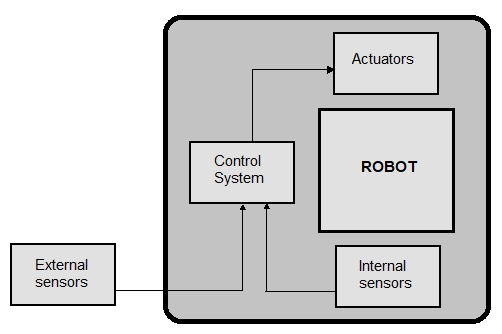Figure 1.3: Schema of robot parts

To get a better understanding of the preceding schema, we are going to see how each component would work in a simulated situation. Imagine a robot that has been ordered to go from point A to point B: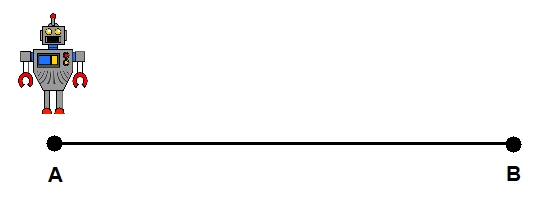Figure 1.4: Robot starting to move from point A

The robot is using a GPS, which is an internal sensor, to constantly check its own position and to check whether it has arrived at the target point. The GPS computes the coordinates and sends them to the control system, which will process them. If the robot hasn't got to point B, the control system tells the actuators to keep going. This situation is represented in the following diagram: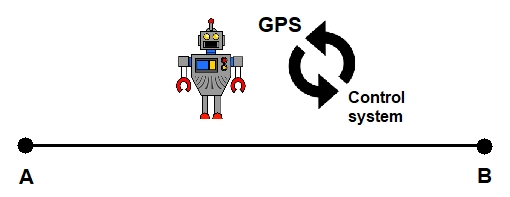Figure 1.5: Robot in the process of completing the path from A to B

On the other hand, if the coordinates sent to the control system by the GPS match the point B, the control system will order the actuators to finish the process, and then the robot won't move: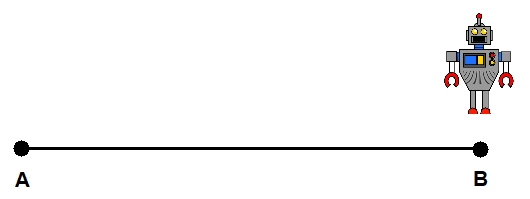Figure 1.6: End of the path! The robot arrives at point B

## Robot Positioning

By using one of the internal sensors mentioned in the preceding section, we can calculate the position of a robot after a certain amount of displacement. This kind of calculation is called odometry and can be performed with the help of the encoders and the information they provide. When discussing this technique, it's important to keep in mind the main advantage and disadvantage:

• Advantage: It can be used to compute the robot's position without external sensors, which would result in a robot's design being much cheaper.

• Disadvantage: The final position calculation is not completely accurate because it depends on the state of the ground and wheels.

Now, let's see how to perform this kind of calculation step by step. Supposing we have a robot that moves on two wheels, we would proceed as follows:

1. First, we should compute the distance completed by the wheels, which is done by using the information extracted from the engine's encoders. In a two-wheeled robot, a simple schema could be like this: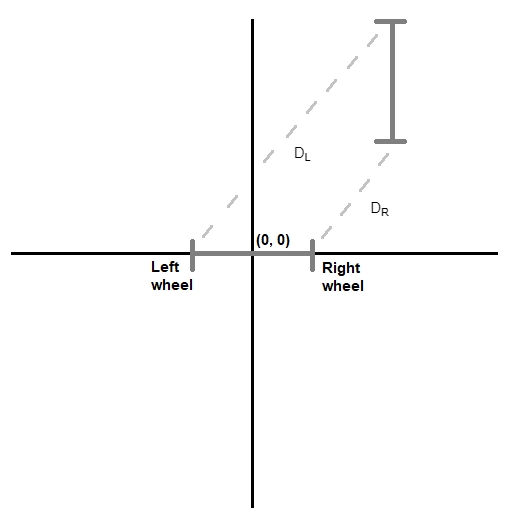Figure 1.7: Schema of a two-wheeled robot's movement

The distance traveled by the left wheel is the dotted line in Figure 1.6 tagged with DL, and DR represents the right wheel.

2. To calculate the linear displacement of the center point of the wheel's axis, we will need the information calculated in the first step. Using the same simple schema, Dc would be the distance:

### Note

If you were working with multi-axial wheels, you should study how the axes are distributed first and then compute the distance traveled by each axis.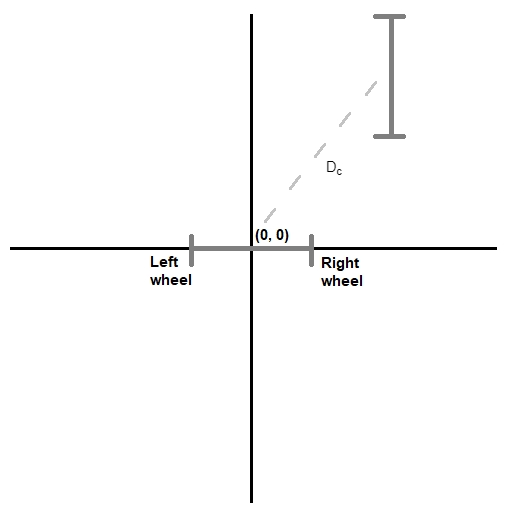Figure 1.8: Schema of a two-wheeled robot's movement (2)

3. To calculate the robot's rotation angle, we will need the final calculation obtained in the first step. The angle named Î± is the one we are referring to: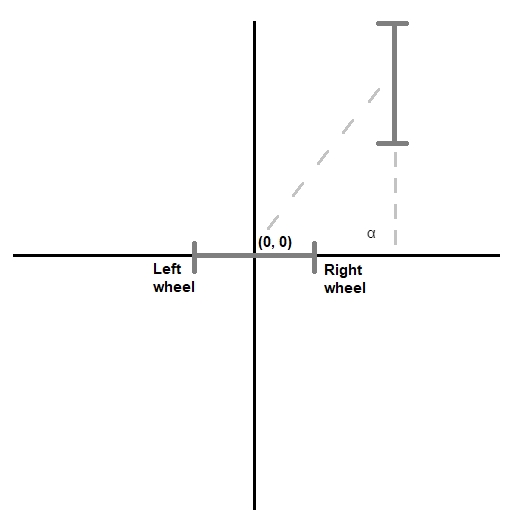Figure 1.9: Schema of a two-wheeled robot's movement (3)

As shown in the diagram, Î± would be 90Âº in this case, which means that the robot has rotated a specific number of degrees.

4. Once you've obtained all the information, it is possible to perform a set of calculations (which will be covered in the next section) to obtain the coordinates of the final position.

### Exercise 1: Computing a Robot's Position

In this exercise, we are using the previous process to compute the position of a two-wheeled robot after it has moved for a certain amount of time. First, let's consider the following data:

• Wheel diameter = 10 cm

• Robot base length = 80 cm

• Encoder counts per lap = 76

• Left encoder counts per 5 seconds = 600

• Right encoder counts per 5 seconds = 900

• Initial position = (0, 0, 0)

• Moving time = 5 seconds

### Note

Encoder counts per lap is the measurement unit that we use to compute the amount of energy generated by an encoder after one lap on its axis. For example, in the information provided above we have the left encoder, which completes 600 counts in 5 seconds. We also know that an encoder needs 76 counts to complete a lap. So, we can deduce that, in 5 seconds, the encoder will complete 7 laps (600/76). This way, if we would know the energy generated by 1 lap, we know the energy generated in 5 seconds.

For the initial position, the first and second numbers refer to the X and Y coordinates, and the last number refers to the rotation angle of the robot. This data is a bit relative, as you have to imagine where the axes begin.

1. Let's compute the completed distance of each wheel. We first compute the number of counts that each encoder performs during the time it moves. This can be easily computed by dividing the total movement by the given encoder time and multiplying it by the number of counts of each encoder:

(Moving time / Encoder time) * Left encoder counts:

(5 / 5) * 600 = 600 counts

(Moving time / Encoder time) * Right encoder counts:

(5 / 5) * 900 = 900 counts

Once this has been calculated, we can use this data to obtain the total distance. As wheels are circular, we can compute each wheel's completed distance as follows:

[2Ï€r / Encoder counts per lap] * Total left encoder counts:

(10Ï€/76) * 600 = 248.02 cm

[2Ï€r / Encoder counts per lap] * Total right encoder counts:

(10Ï€/76) * 900 = 372.03 cm

2. Now compute the linear displacement of the center point of the wheels' axis. This can be done with a simple calculation:

(Left wheel distance + Right wheel distance) / 2:

(248.02 + 372.03) / 2 = 310.03 cm

3. Compute the robot's rotation angle. To do this, you can calculate the difference between the distance completed by each wheel and divide it by the base length:

(Right wheel distance â€“ Left wheel distance) / Base length:

(372.03 - 248.02) / 80 = 1.55 radians

4. Finally, we can compute the final position by calculating each component separately. These are the equations to use to obtain each component:

Final x position = initial x position + (wheels' axis displacement * rotation angle cosine):

0 + (310.03 * cos (1.55)) = 6.45

Final y position = initial y position + (wheels' axis displacement * rotation angle cosine):

0 + (310.03 * sin (1.55)) = 309.96

Final robot rotation = initial robot rotation + robot rotation angle:

0 + 1.55= 1.55

So, after this process, we can conclude that the robot has moved from (0, 0, 0) to (6.45, 309.96, 1.55).

### How to Work with Robots

Like any other software development, the process of implementing applications and programs for robots can be done many different ways.

In the upcoming chapters, we will use frameworks and technologies that make it possible to abstract a specific problem and develop a solution that is easily adaptable to all kinds of robots and devices. In this book, we will be using Robot Operating System (ROS) for this purpose.

Another issue to consider before we start working with robots is the programming language to use. You surely know and have used some languages, but which one is the most appropriate? The real answer to this question is that there is no specific language; it always depends on the problem at hand. But during our book, and due to the kinds of activities that we will work on, we are going to use Python, which, as you may know, is an interpreted, high-level, general-purpose programming language that is used in AI and robotics.

By using Python, as with other languages, you can develop any functionality you want your robot to have. For example, you could give your robot the simple behavior of greeting when it detects a person. You could also program a more complex functionality, for example, to dance when it â€œhearsâ€ music.

Now we are going to go through some exercises and activities that will introduce you to Python for robotics, if you haven't used it before.

### Exercise 2: Computing the Distance Traveled by a Wheel with Python

In this exercise, we are going to implement a simple Python function for computing the distance covered by a wheel using the same process that we performed in Exercise 1, Computing a Robot's Position. These are the steps to be followed:

1. Import the required resources. In this case, we are going to use the number Ï€:

`from math import pi`
2. Create the function with the parameters. To compute this distance, we will need the following:

Wheel diameter in centimeters

Encoder counts per lap

Number of seconds used to measure encoders' counts

Wheel encoder counts during the given number of seconds

Total time of movement

This is the function definition:

`def wheel_distance(diameter, encoder, encoder_time, wheel, movement_time):`
3. Begin with the implementation of the function. First, compute the distance measured by the encoder:

```time = movement_time / encoder_time
wheel_encoder = wheel * time```
4. Transform the obtained distance from above to the one we expect, which would be the distance traveled by the wheel:

`wheel_distance = (wheel_encoder * diameter * pi) / encoder`
5. Return the final value:

`return wheel_distance`
6. You can finally check whether the function is correctly implemented by passing values to it and make the corresponding calculation manual:

`wheel_distance(10, 76, 5, 400, 5)`

This function call should return 165.34698176788385.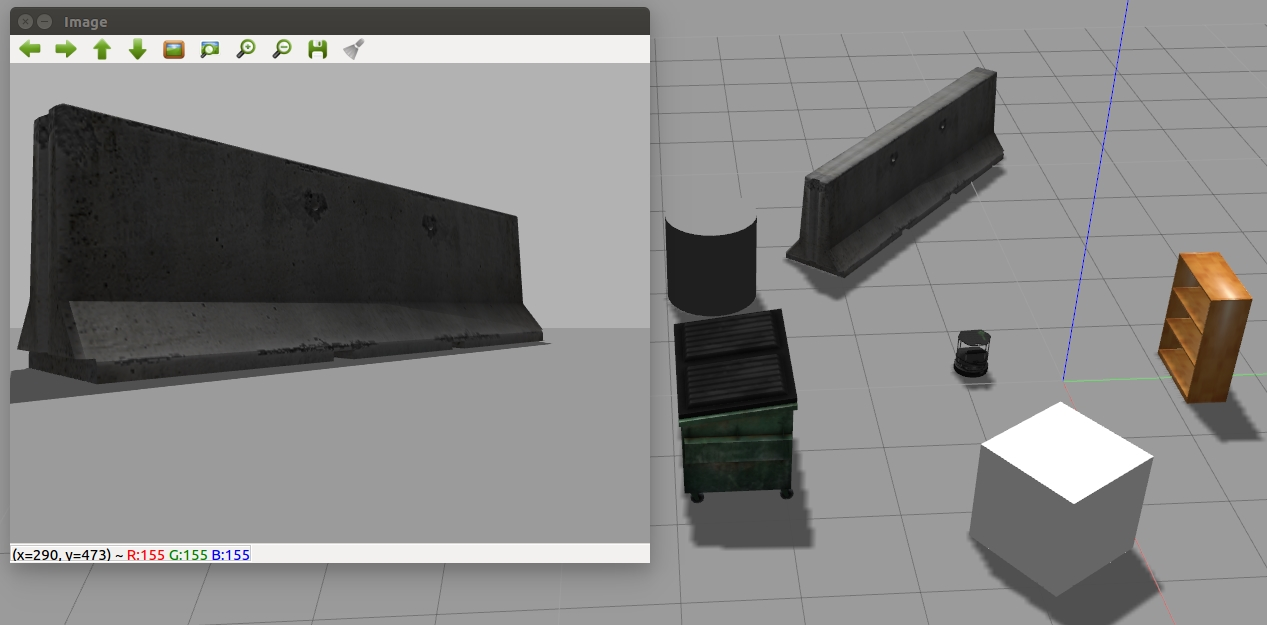Figure 1.10: Final distance covered by the wheel

The output in your notebook should look like this:

### Exercise 3: Computing Final Position with Python

In this exercise, we use Python to compute the final position of a robot, given its initial position, its distance completed by the axis, and its rotation angle. You can do it by following this process:

1. Import the sine and cosine functions:

`from math import cos, sin`
2. Define the function with the required parameters:

The robot's initial position (coordinates)

The completed distance by the robot's central axis

The angle variation from its initial point:

`def final_position(initial_pos, wheel_axis, angle):`

Set a function by coding the formulas used in Exercise 1: Computing a Robot's Position.

They can be coded like this:

```final_x = initial_pos + (wheel_axis * cos(angle))
final_y = initial_pos + (wheel_axis * sin(angle))
final_angle = initial_pos + angle```

### Note

As you may guess by observing this implementation, the initial position has been implemented using a tuple, where the first element matches the â€œXâ€, the second with the â€œYâ€, and the last with the initial angle.

Return the final value by creating a new tuple with the results:

`return(final_x, final_y, final_angle)`
3. Again, you can test the function by calling it with all the arguments and computing the result by hand:

`final_position((0,0,0), 125, 1)`

The preceding code returns the following result:

`(67.53778823351747, 105.18387310098706, 1)`

Here, you can see the whole implementation and an example of a function call: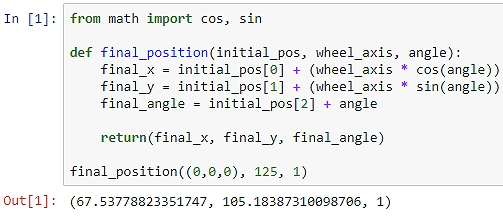Figure 1.11: Final position of the robot computed

### Activity 1: Robot Positioning Using Odometry with Python

You are creating a system that detects the position of a robot after moving for a certain amount of time. Develop a Python function that gives you the final position of a robot after receiving the following data:

• Wheels diameter in centimeters = 10 cm

• Robot base length = 80 cm

• Encoders counts per lap = 76

• Number of seconds used to measure encoders' counts = 600

• Left and right encoder counts during the given number of seconds = 900

• Initial position = (0, 0, 0)

• Movement duration in seconds = 5 seconds

### Note

The functions implemented in the previous exercises can help you to complete the activity. There are a few steps that you can use to proceed ahead with this activity.

1. First, you need to compute the distance completed by each wheel.

2. To move on, you need to calculate the distance completed by the axis.

3. Now compute the robot's rotation angle.

4. Then calculate the final position of the robot.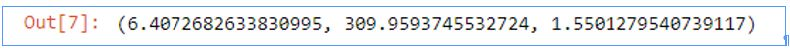Fig 1.12: Final position of a robot computed with the activity's Python function

The output would look like this:

### Note

The solution for this activity can be found on page 248.

## Summary

In this chapter, you have been introduced to the world of robotics. You have learned about advanced techniques, such as NLP and computer vision, combined with robotics. In this chapter, you have also worked with Python, which you will use in the chapters ahead.

In addition, you have made use of odometry to compute a robot's position without external sensors. As you can see, it is not hard to compute a robot's position if the data required is available. Notice that although odometry is a good technique, in future chapters we will use other methods, which will allow us to work with sensors, and that may be more accurate in terms of results.

In the following chapter, we will look at computer vision and work on more practical topics. For example, you will be introduced to machine learning, decision trees, and artificial neural networks, with the goal of applying them to computer vision. You will use them all during the rest of the book, and you will surely get the chance to use them for personal or professional purposes.

• ##### Álvaro Morena Alberola

Álvaro Morena Alberola is a computer engineer and loves robotics and artificial intelligence. Currently, he is working as a software developer. He is extremely interested in the core part of AI, which is based on artificial vision. Álvaro likes working with new technologies and learning how to use advanced tools. He perceives robotics as a way of easing human lives; a way of helping people perform tasks that they cannot do on their own.

Browse publications by this author
• ##### Gonzalo Molina Gallego

Gonzalo Molina Gallego is a computer science graduate and specializes in artificial intelligence and natural language processing. He has experience of working on text-based dialog systems, creating conversational agents, and advising good methodologies. Currently, he is researching new techniques on hybrid-domain conversational systems. Gonzalo thinks that conversational user interfaces are the future.

Browse publications by this author
• ##### Unai Garay Maestre

Unai Garay Maestre is a computer science graduate and specializes in the field of artificial intelligence and computer vision. He successfully contributed to the CIARP conference of 2018 with a paper that takes a new approach to data augmentation using variational autoencoders. He also works as a machine learning developer using deep neural networks applied to images.

Browse publications by this author